CGAL 4.7 - Polygon Mesh Processing
User Manual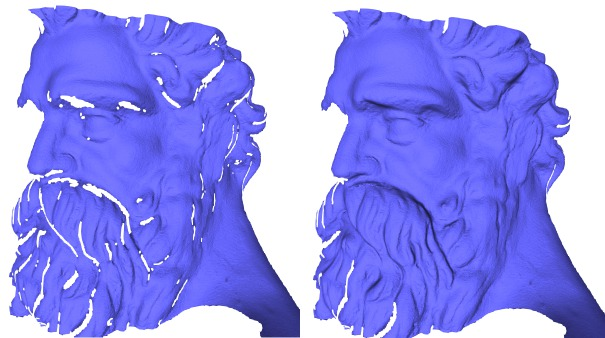# Introduction

This package implements a collection of methods and classes for polygon mesh processing, ranging from basic operations on simplices, to complex geometry processing algorithms. The implementation of this package mainly follows algorithms and references given in Botsch et al.'s book on polygon mesh processing .

## Polygon Mesh

A polygon mesh is a consistent and orientable surface mesh, that can have one or more boundaries. The faces are simple polygons. The edges are segments. Each edge connects two vertices, and is shared by two faces (including the null face for boundary edges). A polygon mesh can have any number of connected components, and also some self-intersections. In this package, a polygon mesh is considered to have the topology of a 2-manifold.

## API

This package follows the BGL API described in chapterBGL. It can thus be used either with Polyhedron_3, Surface_mesh, or any class model of the concept FaceGraph. Each function or class of this package details the requirements on the input polygon mesh.

BGL Named Parameters are used to deal with optional parameters.

## Outline

The algorithms described in this manual are organized in sections. Section 2 describes Meshing algorithms, including triangulation of non-triangulated meshes, refinement and optimization by fairing of triangulated surface meshes. Section 3 lists the available, Hole Filling algorithms, which can possibly be combined with refinement and fairing. Section 4 gives a list of Predicates that can be evaluated on the processed polygon mesh, including point location and self intersection tests. Tools for checking or fixing the Orientation are then described. Section 5 deals with Combinatorial Repairing of polygon meshes and polygon soups. Section 6 offers algorithms for Computing Normals at vertices and on faces of a polygon mesh. Section 7 describes a class that provides an operator able to compute intersections of the a polygon mesh with arbitrary planes, called Slicer. Section 8 deals with methods to compute Connected Components of a polygon mesh, and discard the smallest ones.

# Meshing

A surface patch can be refined by inserting new vertices and flipping edges to get a triangulation. Using a criterion presented in , the density of triangles near the boundary of the patch is approximated by the refinement function. The validity of the mesh is enforced by flipping edges. An edge is flipped only if the opposite edge does not exist in the original mesh and if no degenerate triangles are generated.

A region of the surface mesh (e.g. the refined region), can be faired to obtain a tangentially continuous and smooth surface patch. The region to be faired is defined as a range of vertices that are relocated. The fairing step minimizes a linear bi-Laplacian system with boundary constraints, described in . The visual results of aforementioned steps are depicted by Figure 57.1 (c and d).

## API

Refinement and fairing functions can be applied to an arbitrary region on a polygon mesh, using :

• CGAL::Polygon_mesh_processing::refine() : given a range of facets on a mesh, refines the region.
• CGAL::Polygon_mesh_processing::fair() : given a range of vertices on a mesh, fairs the region.

Fairing needs a sparse linear solver and we recommend the use of Eigen 3.2 or later. Note that fairing might fail if fixed vertices, which are used as boundary conditions, do not suffice to solve the constructed linear system.

Many algorithms require as input meshes in which all the faces have the same degree, or even are triangles. Hence, one may want to triangulate all polygon faces of a mesh.

This package provides the function CGAL::Polygon_mesh_processing::triangulate_faces() that triangulates all faces of the input polygon mesh. An approximated support plane is chosen for each face, orthogonal to the normal vector computed by CGAL::Polygon_mesh_processing::compute_face_normal(). Then, the triangulation of each face is the one obtained by building a CGAL::Constrained_Delaunay_triangulation_2 in this plane. This choice is made because the constrained Delaunay triangulation is the triangulation that, given the edges of the face to be triangulated, maximizes the minimum angle of all the angles of the triangles in the triangulation.

## Meshing Examples

### Refine and Fair a Region on a Polygon Mesh

The following example calls the functions CGAL::Polygon_mesh_processing::refine() and CGAL::Polygon_mesh_processing::fair() for some selected regions on the input polygon mesh.

#include <CGAL/Exact_predicates_inexact_constructions_kernel.h>
#include <CGAL/Polyhedron_3.h>
#include <CGAL/boost/graph/graph_traits_Polyhedron_3.h>
#include <CGAL/IO/Polyhedron_iostream.h>
#include <CGAL/Polygon_mesh_processing/refine.h>
#include <CGAL/Polygon_mesh_processing/fair.h>
#include <fstream>
#include <map>
typedef CGAL::Polyhedron_3<Kernel> Polyhedron;
typedef Polyhedron::Vertex_handle Vertex_handle;
// extract vertices which are at most k (inclusive)
// far from vertex v in the graph of edges
void extract_k_ring(Vertex_handle v,
int k,
std::vector<Vertex_handle>& qv)
{
std::map<Vertex_handle, int> D;
qv.push_back(v);
D[v] = 0;
std::size_t current_index = 0;
int dist_v;
while (current_index < qv.size() && (dist_v = D[qv[current_index]]) < k)
{
v = qv[current_index++];
Polyhedron::Halfedge_around_vertex_circulator e(v->vertex_begin()), e_end(e);
do {
Vertex_handle new_v = e->opposite()->vertex();
if (D.insert(std::make_pair(new_v, dist_v + 1)).second) {
qv.push_back(new_v);
}
} while (++e != e_end);
}
}
int main(int argc, char* argv[])
{
const char* filename = (argc > 1) ? argv : "data/blobby.off";
std::ifstream input(filename);
Polyhedron poly;
if ( !input || !(input >> poly) || poly.empty() ) {
std::cerr << "Not a valid off file." << std::endl;
return 1;
}
std::vector<Polyhedron::Facet_handle> new_facets;
std::vector<Vertex_handle> new_vertices;
faces(poly),
std::back_inserter(new_facets),
std::back_inserter(new_vertices),
CGAL::Polygon_mesh_processing::parameters::density_control_factor(2.));
std::ofstream refined_off("refined.off");
refined_off << poly;
refined_off.close();
std::cout << "Refinement added " << new_vertices.size() << " vertices." << std::endl;
Polyhedron::Vertex_iterator v = poly.vertices_begin();
std::advance(v, 82/*e.g.*/);
std::vector<Vertex_handle> region;
extract_k_ring(v, 12/*e.g.*/, region);
bool success = CGAL::Polygon_mesh_processing::fair(poly, region);
std::cout << "Fairing : " << (success ? "succeeded" : "failed") << std::endl;
std::ofstream faired_off("faired.off");
faired_off << poly;
faired_off.close();
return 0;
}

### Triangulate a Polygon Mesh

Triangulating a polygon mesh can be achieved through the function CGAL::Polygon_mesh_processing::triangulate_faces() as shown in the following example.

#include <CGAL/Exact_predicates_inexact_constructions_kernel.h>
#include <CGAL/Surface_mesh.h>
#include <CGAL/boost/graph/graph_traits_Surface_mesh.h>
#include <CGAL/Polygon_mesh_processing/triangulate_faces.h>
#include <fstream>
typedef Kernel::Point_3 Point;
typedef CGAL::Surface_mesh<Point> Surface_mesh;
int main(int argc, char* argv[])
{
const char* filename = (argc > 1) ? argv : "data/P.off";
std::ifstream input(filename);
Surface_mesh mesh;
if (!input || !(input >> mesh) || mesh.is_empty())
{
std::cerr << "Not a valid off file." << std::endl;
return 1;
}
std::ofstream cube_off("P_tri.off");
cube_off << mesh;
cube_off.close();
return 0;
}

# Hole Filling

This package provides an algorithm for filling one closed hole that is either in a triangulated surface mesh or defined by a sequence of points that describe a polyline. The main steps of the algorithm are described in  and can be summarized as follows.

First, the largest patch triangulating the boundary of the hole is generated without introducing any new vertex. The patch is selected so as to minimize a quality function evaluated for all possible triangular patches. The quality function first minimizes the worst dihedral angle between patch triangles, then the total surface area of the patch as a tiebreaker. Following the suggestions in , the performance of the algorithm is significantly improved by narrowing the search space to faces of a 3D Delaunay triangulation of the hole boundary vertices, from all possible patches, while searching for the best patch with respect to the aforementioned quality criteria.

For some complicated input hole boundary, the generated patch may have self-intersections. After hole filling, the generated patch can be refined and faired using the meshing functions CGAL::Polygon_mesh_processing::refine() and CGAL::Polygon_mesh_processing::fair() described in Section Meshing.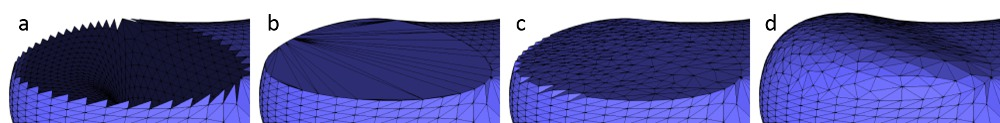Figure 57.1 Results of the main steps of the algorithm. From left to right: (a) the hole, (b) the hole after its triangulation, (c) after triangulation and refinement, (d) after triangulation, refinement and fairing.

## API

This package provides four functions for hole filling:

• triangulate_hole_polyline() : given a sequence of points defining the hole, triangulates the hole.
• triangulate_hole() : given a border halfedge on the boundary of the hole on a mesh, triangulates the hole.
• triangulate_and_refine_hole() : in addition to triangulate_hole() the generated patch is refined.
• triangulate_refine_and_fair_hole() : in addition to triangulate_and_refine_hole() the generated patch is also faired.

## Examples

### Triangulate a Polyline

The following example triangulates a hole described by an input polyline.

#include <CGAL/Exact_predicates_inexact_constructions_kernel.h>
#include <CGAL/Polygon_mesh_processing/triangulate_hole.h>
#include <CGAL/utility.h>
#include <vector>
#include <iterator>
typedef Kernel::Point_3 Point;
int main()
{
std::vector<Point> polyline;
polyline.push_back(Point( 1.,0.,0.));
polyline.push_back(Point( 0.,1.,0.));
polyline.push_back(Point(-1.,0.,0.));
polyline.push_back(Point( 1.,1.,0.));
// repeating first point (i.e. polyline.push_back(Point(1.,0.,0.)) ) is optional
// any type, having Type(int, int, int) constructor available, can be used to hold output triangles
typedef CGAL::Triple<int, int, int> Triangle_int;
std::vector<Triangle_int> patch;
patch.reserve(polyline.size() -2); // there will be exactly n-2 triangles in the patch
polyline,
std::back_inserter(patch));
for(std::size_t i = 0; i < patch.size(); ++i)
{
std::cout << "Triangle " << i << ": "
<< patch[i].first << " " << patch[i].second << " " << patch[i].third
<< std::endl;
}
// note that no degenerate triangles are generated in the patch
std::vector<Point> polyline_collinear;
polyline_collinear.push_back(Point(1.,0.,0.));
polyline_collinear.push_back(Point(2.,0.,0.));
polyline_collinear.push_back(Point(3.,0.,0.));
polyline_collinear.push_back(Point(4.,0.,0.));
std::vector<Triangle_int> patch_will_be_empty;
polyline_collinear,
back_inserter(patch_will_be_empty));
CGAL_assertion(patch_will_be_empty.empty());
return 0;
}

### Hole Filling From the Border of the Hole

If the input polygon mesh has a hole or more than one hole, it is possible to iteratively fill them by detecting border edges (i.e. with only one incident non-null face) after each hole filling step.

Holes are filled one after the other, and the process stops when there is no border edge left.

This process is illustrated by the example below, where holes are iteratively filled, refined and faired to get a faired mesh with no hole.

#include <CGAL/Exact_predicates_inexact_constructions_kernel.h>
#include <CGAL/Polyhedron_3.h>
#include <CGAL/IO/Polyhedron_iostream.h>
#include <CGAL/boost/graph/graph_traits_Polyhedron_3.h>
#include <CGAL/Polygon_mesh_processing/triangulate_hole.h>
#include <iostream>
#include <fstream>
#include <vector>
#include <boost/foreach.hpp>
typedef CGAL::Polyhedron_3<Kernel> Polyhedron;
typedef Polyhedron::Halfedge_handle Halfedge_handle;
typedef Polyhedron::Facet_handle Facet_handle;
typedef Polyhedron::Vertex_handle Vertex_handle;
int main(int argc, char* argv[])
{
const char* filename = (argc > 1) ? argv : "data/mech-holes-shark.off";
std::ifstream input(filename);
Polyhedron poly;
if ( !input || !(input >> poly) || poly.empty() ) {
std::cerr << "Not a valid off file." << std::endl;
return 1;
}
// Incrementally fill the holes
unsigned int nb_holes = 0;
BOOST_FOREACH(Halfedge_handle h, halfedges(poly))
{
if(h->is_border())
{
std::vector<Facet_handle> patch_facets;
std::vector<Vertex_handle> patch_vertices;
bool success = CGAL::cpp11::get<0>(
poly,
h,
std::back_inserter(patch_facets),
std::back_inserter(patch_vertices),
CGAL::Polygon_mesh_processing::parameters::vertex_point_map(get(CGAL::vertex_point, poly)).
geom_traits(Kernel())) );
std::cout << " Number of facets in constructed patch: " << patch_facets.size() << std::endl;
std::cout << " Number of vertices in constructed patch: " << patch_vertices.size() << std::endl;
std::cout << " Fairing : " << (success ? "succeeded" : "failed") << std::endl;
++nb_holes;
}
}
std::cout << std::endl;
std::cout << nb_holes << " holes have been filled" << std::endl;
std::ofstream out("filled.off");
out.precision(17);
out << poly << std::endl;
return 0;
}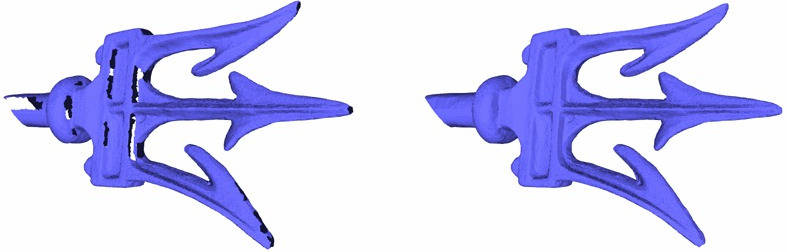Figure 57.2 Holes in the fork model are filled with triangle patches.

# Predicates

This packages provides several predicates to be evaluated with respect to a triangle mesh.

## Self Intersections

Self intersections can be detected from a triangle mesh, by calling the predicate CGAL::Polygon_mesh_processing::does_self_intersect(). Additionally, the function CGAL::Polygon_mesh_processing::self_intersections() records all pairs of intersecting triangles.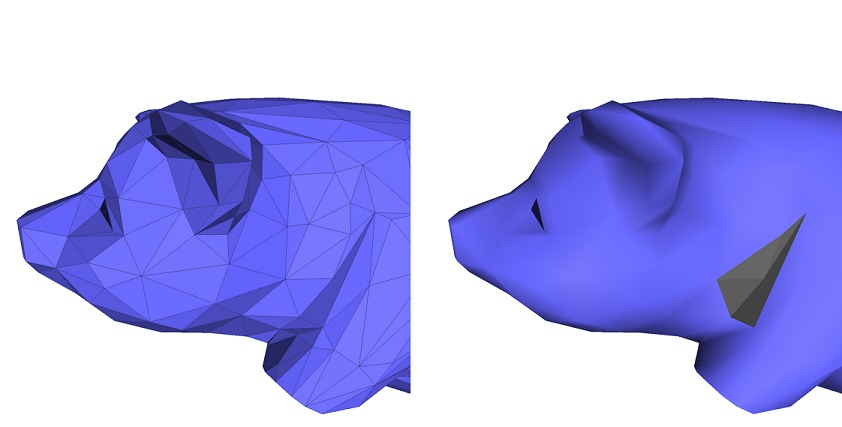Figure 57.3 Detecting self-intersections on a triangle mesh. The intersecting triangles are displayed in dark grey on the right image.

### Self Intersections Example

#include <CGAL/Exact_predicates_inexact_constructions_kernel.h>
#include <CGAL/Surface_mesh.h>
#include <CGAL/boost/graph/graph_traits_Surface_mesh.h>
#include <CGAL/Polygon_mesh_processing/self_intersections.h>
#include <fstream>
typedef boost::graph_traits<Mesh>::face_descriptor face_descriptor;
namespace PMP = CGAL::Polygon_mesh_processing;
int main(int argc, char* argv[])
{
const char* filename = (argc > 1) ? argv : "data/pig.off";
std::ifstream input(filename);
Mesh mesh;
if (!input || !(input >> mesh))
{
std::cerr << "Not a valid off file." << std::endl;
return 1;
}
bool intersecting = PMP::does_self_intersect(mesh,
PMP::parameters::vertex_point_map(get(CGAL::vertex_point, mesh)));
std::cout
<< (intersecting ? "There are self-intersections." : "There is no self-intersection.")
<< std::endl;
std::vector<std::pair<face_descriptor, face_descriptor> > intersected_tris;
std::back_inserter(intersected_tris),
PMP::parameters::vertex_point_map(get(CGAL::vertex_point, mesh)));
std::cout << intersected_tris.size() << " pairs of triangles intersect." << std::endl;
return 0;
}

## Side of Triangle Mesh

The class CGAL::Side_of_triangle_mesh provides a functor that tests whether a query point is inside, outside, or on the boundary of the domain bounded by a given closed triangle mesh.

A point is said to be on the bounded side of the domain bounded by the input triangle mesh if an odd number of surfaces is crossed when walking from the point to infinity. The input triangle mesh is expected to contain no self-intersections and to be free from self-inclusions.

The algorithm can handle the case of a triangle mesh with several connected components, and returns correct results. In case of self-inclusions, the ray intersections parity test is performed, and the execution will not fail. However, the user should be aware that the predicate alternately considers sub-volumes to be on the bounded and unbounded sides of the input triangle mesh.

### Inside Test Example

#include <CGAL/Exact_predicates_inexact_constructions_kernel.h>
#include <CGAL/Polyhedron_3.h>
#include <CGAL/IO/Polyhedron_iostream.h>
#include <CGAL/boost/graph/graph_traits_Polyhedron_3.h>
#include <CGAL/point_generators_3.h>
#include <CGAL/Side_of_triangle_mesh.h>
#include <vector>
#include <fstream>
#include <limits>
#include <boost/foreach.hpp>
typedef K::Point_3 Point;
typedef CGAL::Polyhedron_3<K> Polyhedron;
double max_coordinate(const Polyhedron& poly)
{
double max_coord = (std::numeric_limits<double>::min)();
BOOST_FOREACH(Polyhedron::Vertex_handle v, vertices(poly))
{
Point p = v->point();
max_coord = (std::max)(max_coord, p.x());
max_coord = (std::max)(max_coord, p.y());
max_coord = (std::max)(max_coord, p.z());
}
return max_coord;
}
int main(int argc, char* argv[])
{
const char* filename = (argc > 1) ? argv : "data/eight.off";
std::ifstream input(filename);
Polyhedron poly;
if (!input || !(input >> poly) || poly.empty())
{
std::cerr << "Not a valid off file." << std::endl;
return 1;
}
double size = max_coordinate(poly);
unsigned int nb_points = 100;
std::vector<Point> points;
points.reserve(nb_points);
CGAL::Random_points_in_cube_3<Point> gen(size);
for (unsigned int i = 0; i < nb_points; ++i)
points.push_back(*gen++);
std::cout << "Test " << nb_points << " random points in cube "
<< "[-" << size << "; " << size <<"]" << std::endl;
int nb_inside = 0;
int nb_boundary = 0;
for (std::size_t i = 0; i < nb_points; ++i)
{
CGAL::Bounded_side res = inside(points[i]);
if (res == CGAL::ON_BOUNDED_SIDE) { ++nb_inside; }
if (res == CGAL::ON_BOUNDARY) { ++nb_boundary; }
}
std::cerr << "Total query size: " << points.size() << std::endl;
std::cerr << " " << nb_inside << " points inside " << std::endl;
std::cerr << " " << nb_boundary << " points on boundary " << std::endl;
std::cerr << " " << points.size() - nb_inside - nb_boundary << " points outside " << std::endl;
return 0;
}

# Orientation

This package provides functions dealing with the orientation of faces in a closed polygon mesh.

The function CGAL::Polygon_mesh_processing::is_outward_oriented() checks whether an oriented polygon mesh is oriented such that the normals to all faces are oriented towards the outside of the domain bounded by the input polygon mesh.

The function CGAL::Polygon_mesh_processing::reverse_face_orientations() reverses the orientation of halfedges around faces. As a consequence, the normal computed for each face (see Section Computing Normals) is also reversed.

The Polygon Soup Example puts these functions at work on a polygon soup.

# Combinatorial Repairing

## Stitching

It happens that a polygon mesh has several edges and vertices that are duplicated. For those edges and vertices, the connectivity of the mesh is incomplete, if not considered incorrect.

Stitching the borders of such a polygon mesh consists in two main steps. First, border edges that are similar but duplicated are detected and paired. Then, they are "stitched" together so that the edges and vertices duplicates are removed from the mesh, and each of these remaining edges is incident to exactly two faces.

The function CGAL::Polygon_mesh_processing::stitch_borders() performs such repairing operation. The input mesh should be manifold. Otherwise, stitching is not guaranteed to succeed.

### Stitching Example

The following example applies the stitching operation to a simple quad mesh with duplicated border edges.

#include <CGAL/Exact_predicates_inexact_constructions_kernel.h>
#include <CGAL/Polyhedron_3.h>
#include <CGAL/IO/Polyhedron_iostream.h>
#include <CGAL/Polygon_mesh_processing/stitch_borders.h>
#include <iostream>
#include <fstream>
typedef CGAL::Polyhedron_3<K> Polyhedron;
int main(int argc, char* argv[])
{
const char* filename = (argc > 1) ? argv : "data/full_border_quads.off";
std::ifstream input(filename);
Polyhedron mesh;
if (!input || !(input >> mesh) || mesh.is_empty()) {
std::cerr << "Not a valid off file." << std::endl;
return 1;
}
std::cout << "Before stitching : " << std::endl;
std::cout << "\t Number of vertices :\t" << mesh.size_of_vertices() << std::endl;
std::cout << "\t Number of halfedges :\t" << mesh.size_of_halfedges() << std::endl;
std::cout << "\t Number of facets :\t" << mesh.size_of_facets() << std::endl;
std::cout << "Stitching done : " << std::endl;
std::cout << "\t Number of vertices :\t" << mesh.size_of_vertices() << std::endl;
std::cout << "\t Number of halfedges :\t" << mesh.size_of_halfedges() << std::endl;
std::cout << "\t Number of facets :\t" << mesh.size_of_facets() << std::endl;
return 0;
}

## Polygon Soups

When the faces of a polygon mesh are given but the connectivity is unknown, we must deal with of a polygon soup.

Before running any of the algorithms on the so-called polygon soup, one should ensure that the polygons are consistently oriented. To do so, this package provides the function CGAL::Polygon_mesh_processing::orient_polygon_soup(), described in .

To deal with polygon soups that cannot be converted to a combinatorial manifold surface, some points are duplicated. Because a polygon soup does not have any connectivity (each point has as many occurences as the number of polygons it belongs to), duplicating one point (or a pair of points) amounts to duplicate the polygon to which it belongs.

The duplicated points are either an endpoint of an edge incident to more than two polygons, an endpoint of an edge between two polygons with incompatible orientations (during the re-orientation process), or more generally a point p at which the intersection of an infinitesimally small ball centered at p with the polygons incident to it is not a topological disk.

Once the polygon soup is consistently oriented, with possibly duplicated (or more) points, the connectivity can be recovered and made consistent to build a valid polygon mesh. The function CGAL::Polygon_mesh_processing::polygon_soup_to_polygon_mesh() performs this mesh construction step.

### Polygon Soup Example

This example shows how to generate a mesh from a polygon soup. The first step is to get a soup of consistently oriented faces, before rebuilding the connectivity. In this example, some orientation tests are performed on the output polygon mesh to illustrate Section Orientation.

#include <CGAL/Exact_predicates_inexact_constructions_kernel.h>
#include <CGAL/Polyhedron_3.h>
#include <CGAL/IO/Polyhedron_iostream.h>
#include <CGAL/IO/OFF_reader.h>
#include <CGAL/Polygon_mesh_processing/orient_polygon_soup.h>
#include <CGAL/Polygon_mesh_processing/polygon_soup_to_polygon_mesh.h>
#include <CGAL/Polygon_mesh_processing/orientation.h>
#include <vector>
#include <fstream>
#include <iostream>
typedef CGAL::Polyhedron_3<K> Polyhedron;
int main(int argc, char* argv[])
{
const char* filename = (argc > 1) ? argv : "data/tet-shuffled.off";
std::ifstream input(filename);
if (!input)
{
std::cerr << "Cannot open file " << std::endl;
return 1;
}
std::vector<K::Point_3> points;
std::vector< std::vector<std::size_t> > polygons;
if (!CGAL::read_OFF(input, points, polygons))
{
std::cerr << "Error parsing the OFF file " << std::endl;
return 1;
}
Polyhedron mesh;
std::ofstream out("tet-oriented1.off");
out << mesh;
out.close();
std::ofstream out2("tet-oriented2.off");
out2 << mesh;
out2.close();
return 0;
}

# Computing Normals

This package provides methods to compute normals on the polygon mesh. The normal can either be computed for each single face, or estimated for each vertex, as the average of its incident face normals. These computations are performed with :

• CGAL::Polygon_mesh_processing::compute_face_normal()
• CGAL::Polygon_mesh_processing::compute_vertex_normal()

We further provide functions to compute all the normals to faces, or to vertices, or to both :

• CGAL::Polygon_mesh_processing::compute_face_normals()
• CGAL::Polygon_mesh_processing::compute_vertex_normals()
• CGAL::Polygon_mesh_processing::compute_normals().

Property maps are used to record the computed normals.

## Normals Computation Example

The following example illustrates how to compute the normals to faces and vertices and store them in property maps.

#include <CGAL/Exact_predicates_inexact_constructions_kernel.h>
#include <CGAL/Surface_mesh.h>
#include <CGAL/Polygon_mesh_processing/compute_normal.h>
#include <iostream>
#include <fstream>
typedef K::Point_3 Point;
typedef K::Vector_3 Vector;
typedef CGAL::Surface_mesh<Point> Surface_mesh;
typedef boost::graph_traits<Surface_mesh>::vertex_descriptor vertex_descriptor;
typedef boost::graph_traits<Surface_mesh>::face_descriptor face_descriptor;
int main(int argc, char* argv[])
{
const char* filename = (argc > 1) ? argv : "data/eight.off";
std::ifstream input(filename);
Surface_mesh mesh;
if (!input || !(input >> mesh) || mesh.is_empty()) {
std::cerr << "Not a valid off file." << std::endl;
return 1;
}
Surface_mesh::Property_map<face_descriptor, Vector> fnormals =
mesh.add_property_map<face_descriptor, Vector>
("f:normals", CGAL::NULL_VECTOR).first;
Surface_mesh::Property_map<vertex_descriptor, Vector> vnormals =
mesh.add_property_map<vertex_descriptor, Vector>
("v:normals", CGAL::NULL_VECTOR).first;
vnormals,
fnormals,
CGAL::Polygon_mesh_processing::parameters::vertex_point_map(mesh.points()).
geom_traits(K()));
std::cout << "Face normals :" << std::endl;
BOOST_FOREACH(face_descriptor fd, faces(mesh)){
std::cout << fnormals[fd] << std::endl;
}
std::cout << "Vertex normals :" << std::endl;
BOOST_FOREACH(vertex_descriptor vd, vertices(mesh)){
std::cout << vnormals[vd] << std::endl;
}
return 0;
}

# Slicer

The CGAL::Polygon_mesh_slicer is an operator that intersects a triangle surface mesh with a plane. It records the intersection as a set of polylines since the intersection can be made of more than one connected component. The degenerate case where the intersection is a single point is handled.

Figure 57.4 shows the polylines returned by the slicing operation for a triangle mesh and a set of parallel planes.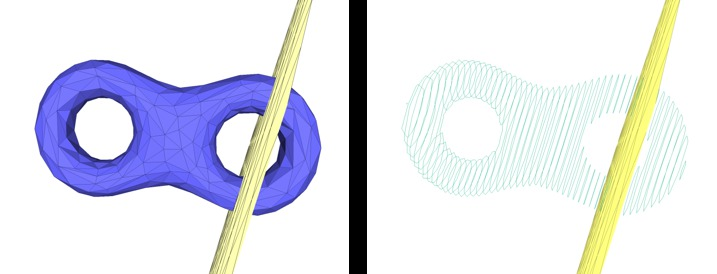Figure 57.4 Slicing a mesh. A triangle mesh (left) and the polylines computed by the mesh slicer by intersecting a set of parallel planes (right).

## Slicer Example

The example below illustrates how to use the mesh slicer for a given triangle mesh and a plane. Two constructors are used in the example for pedagogical purposes.

#include <CGAL/Exact_predicates_inexact_constructions_kernel.h>
#include <CGAL/Surface_mesh.h>
#include <CGAL/boost/graph/graph_traits_Surface_mesh.h>
#include <CGAL/AABB_halfedge_graph_segment_primitive.h>
#include <CGAL/AABB_tree.h>
#include <CGAL/AABB_traits.h>
#include <CGAL/Polygon_mesh_slicer.h>
#include <fstream>
typedef std::vector<K::Point_3> Polyline_type;
typedef std::list< Polyline_type > Polylines;
typedef CGAL::AABB_traits<K, HGSP> AABB_traits;
typedef CGAL::AABB_tree<AABB_traits> AABB_tree;
int main(int argc, char* argv[])
{
const char* filename = (argc > 1) ? argv : "data/eight.off";
std::ifstream input(filename);
Mesh mesh;
if (!input || !(input >> mesh) || mesh.is_empty()) {
std::cerr << "Not a valid off file." << std::endl;
return 1;
}
// Slicer constructor from the mesh
Polylines polylines;
slicer(K::Plane_3(0, 0, 1, -0.4), std::back_inserter(polylines));
std::cout << "At z = 0.4, the slicer intersects "
<< polylines.size() << " polylines" << std::endl;
polylines.clear();
slicer(K::Plane_3(0, 0, 1, 0.2), std::back_inserter(polylines));
std::cout << "At z = -0.2, the slicer intersects "
<< polylines.size() << " polylines" << std::endl;
polylines.clear();
// Use the Slicer constructor from a pre-built AABB_tree
AABB_tree tree(edges(mesh).first, edges(mesh).second, mesh);
CGAL::Polygon_mesh_slicer<Mesh, K> slicer_aabb(mesh, tree);
slicer_aabb(K::Plane_3(0, 0, 1, -0.4), std::back_inserter(polylines));
std::cout << "At z = 0.4, the slicer intersects "
<< polylines.size() << " polylines" << std::endl;
polylines.clear();
return 0;
}

# Connected Components

This package provides functions to enumerate and store the connected components of a polygon mesh. The connected components can be either closed and geometrically separated, or separated by border or user-specified constraint edges.

First, the function CGAL::Polygon_mesh_processing::connected_component() collects all the faces that belong to the same connected component as the face that is given as a parameter.

Then, CGAL::Polygon_mesh_processing::connected_components() collects all the connected components, and fills a property map with the indices of the different connected components.

The functions CGAL::Polygon_mesh_processing::keep_connected_components() and CGAL::Polygon_mesh_processing::remove_connected_components() enable the user to keep and remove only a selection of connected components, provided either as a range of faces that belong to the desired connected components or as a range of connected component ids (one or more per connected component).

Finally, CGAL::Polygon_mesh_processing::keep_largest_connected_components() enables the user to keep only the largest connected components. This feature can for example be useful for noisy data were small connected components should be discarded in favour of major connected components.

## Connected Components Example

The following example shows how to record the connected components of a polygon mesh. In particular, we provide an example for the optional parameter EdgeConstraintMap, a property map that returns information about an edge being a constraint or not. A constraint provides a mean to demarcate the border of a connected component, and prevents the propagation of a connected component index to cross it.

#include <CGAL/Exact_predicates_inexact_constructions_kernel.h>
#include <CGAL/Surface_mesh.h>
#include <CGAL/Polygon_mesh_processing/connected_components.h>
#include <boost/function_output_iterator.hpp>
#include <boost/property_map/property_map.hpp>
#include <boost/foreach.hpp>
#include <iostream>
#include <fstream>
#include <map>
typedef Kernel::Point_3 Point;
typedef Kernel::Compare_dihedral_angle_3 Compare_dihedral_angle_3;
namespace PMP = CGAL::Polygon_mesh_processing;
template <typename G>
struct Constraint : public boost::put_get_helper<bool,Constraint<G> >
{
typedef typename boost::graph_traits<G>::edge_descriptor edge_descriptor;
typedef boost::readable_property_map_tag category;
typedef bool value_type;
typedef bool reference;
typedef edge_descriptor key_type;
Constraint()
:g_(NULL)
{}
Constraint(G& g, double bound)
: g_(&g), bound_(bound)
{}
bool operator[](edge_descriptor e) const
{
const G& g = *g_;
return compare_(g.point(source(e, g)),
g.point(target(e, g)),
g.point(target(next(halfedge(e, g), g), g)),
g.point(target(next(opposite(halfedge(e, g), g), g), g)),
bound_) == CGAL::SMALLER;
}
const G* g_;
Compare_dihedral_angle_3 compare_;
double bound_;
};
template <typename PM>
struct Put_true
{
Put_true(const PM pm)
:pm(pm)
{}
template <typename T>
void operator()(const T& t)
{
put(pm, t, true);
}
PM pm;
};
int main(int argc, char* argv[])
{
const char* filename = (argc > 1) ? argv : "data/blobby_3cc.off";
std::ifstream input(filename);
Mesh mesh;
if (!input || !(input >> mesh) || mesh.is_empty()) {
std::cerr << "Not a valid off file." << std::endl;
return 1;
}
typedef boost::graph_traits<Mesh>::face_descriptor face_descriptor;
const double bound = std::cos(0.75 * CGAL_PI);
std::vector<face_descriptor> cc;
face_descriptor fd = *faces(mesh).first;
mesh,
std::back_inserter(cc));
std::cerr << "Connected components without edge constraints" << std::endl;
std::cerr << cc.size() << " faces in the CC of " << fd << std::endl;
// Instead of writing the faces into a container, you can set a face property to true
typedef Mesh::Property_map<face_descriptor, bool> F_select_map;
F_select_map fselect_map =
mesh.add_property_map<face_descriptor, bool>("f:select", false).first;
mesh,
boost::make_function_output_iterator(Put_true<F_select_map>(fselect_map)));
std::cerr << "\nConnected components with edge constraints (dihedral angle < 3/4 pi)" << std::endl;
Mesh::Property_map<face_descriptor, std::size_t> fccmap =
mesh.add_property_map<face_descriptor, std::size_t>("f:CC").first;
std::size_t num = PMP::connected_components(mesh,
fccmap,
PMP::parameters::edge_is_constrained_map(Constraint<Mesh>(mesh, bound)));
std::cerr << "- The graph has " << num << " connected components (face connectivity)" << std::endl;
typedef std::map<std::size_t/*index of CC*/, unsigned int/*nb*/> Components_size;
Components_size nb_per_cc;
BOOST_FOREACH(face_descriptor f , faces(mesh)){
nb_per_cc[ fccmap[f] ]++;
}
BOOST_FOREACH(const Components_size::value_type& cc, nb_per_cc){
std::cout << "\t CC #" << cc.first
<< " is made of " << cc.second << " faces" << std::endl;
}
std::cerr << "- We keep the two largest components" << std::endl;
2,
PMP::parameters::edge_is_constrained_map(Constraint<Mesh>(mesh, bound)));
return 0;
}

# Requirements Summary

The table below recapitulates the basic requirements of each function of this package. For each requirement, the table answers a question :

• should the input mesh be a pure triangle mesh?
• should the input mesh be self-intersection free?
• should the input mesh be closed (with no boundary)?
Function or Class Pure Triangle Self-Intersection Free Closed
fair() no no no
refine() no no no
triangulate_faces() no no no
triangulate_hole() no no no
triangulate_and_refine_hole() no no no
triangulate_refine_and_fair_hole() no no no
triangulate_hole_polyline() no no no
does_self_intersect() yes no no
self_intersections() yes no no
Side_of_triangle_mesh yes yes yes
is_outward_oriented() no no yes
reverse_face_orientations() no no no
stitch_borders() no no no
polygon_soup_to_polygon_mesh() no no no
orient_polygon_soup() no no no
compute_face_normal() no no no
compute_face_normals() no no no
compute_vertex_normal() no no no
compute_vertex_normals() no no no
compute_normals() no no no
Polygon_mesh_slicer yes yes no
connected_component() no no no
connected_components() no no no
keep_largest_connected_components() no no no
keep_connected_components() no no no
remove_connected_components() no no no

# Implementation History

A first version of this package was started by Ilker O. Yaz and Sébastien Loriot. Jane Tournois worked on the finalization of the API, code, and documentation.